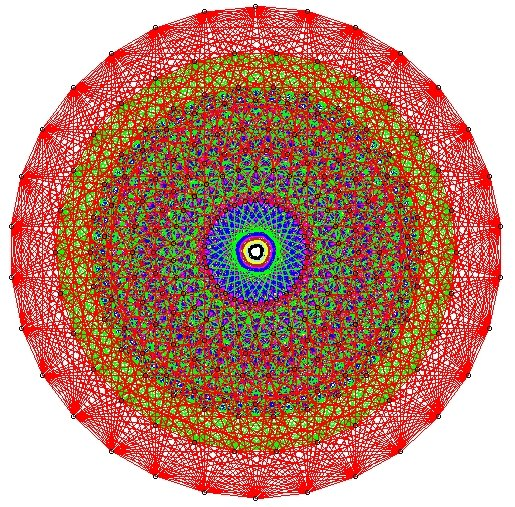Atlas of Lie Groups and RepresentationsGalois Cohomology: code To use this code in realex, save it to a file mygalois.rx, and load it in realex with the command 8) or (rank=5)) then ["A"+rank,"B"+rank,"C"+rank,"D"+rank] elif (rank>5) then ["A"+rank,"B"+rank,"C"+rank,"D"+rank,"E"+rank] else let types_of_given_rank=[["A"],["A","B","G"],["A","B","C"],["A","B","C","D","F"] ] in for type in types_of_given_rank[rank-1] do type+rank od fi {list of allowed inner classes for given simple type} {e.g. all_inner_classes ("A2") Value: ["e","u"]} set all_inner_classes(string type_and_rank)=[string]: let (type,rank)=(type_and_rank,type_and_rank) in if ((type="A" and rank !="1") or (type="D") or (type="E" and rank="6")) then ["e","u"] else ["e"] fi {generate list of all simple groups either simply connected "sc" or adjoint "ad" of given rank e.g. all_simple("sc",2), automatically runs over all inner classes} set all_simple_given_isogeny_and_rank(string isogeny,int rank)= let rootsystems=all_simple_root_systems_given_rank(rank) in let rv=[RealForm]:[] in for rs in rootsystems do let inner_classes=all_inner_classes(rs) in for ic in inner_classes do if (isogeny ="sc") then rv:=rv#real_forms(Cartan_class(inner_class(simply_connected (Lie_type (rs)),ic),0)) elif (isogeny="ad") then rv:=rv#real_forms(Cartan_class(inner_class(adjoint (Lie_type (rs)),ic),0)) fi od od;rv {all_simple("sc",k) or all_simple("ad",k)} set all_simple(string isogeny, int rank)=all_simple_given_isogeny_and_rank(isogeny,rank) set all_simple_simply_connected_rank(int rank)=all_simple("sc",rank) set all_simple_adjoint_rank(int rank)=all_simple("ad",rank) set all_simple_rank(int rank)=all_simple_simply_connected_rank(rank)#all_simple_adjoint_rank(rank) {H^1(\Gamma,G)=H^1(\theta,G) is the number of strong real forms with given x^2\in Z} set H1(RealForm G)=int:#strong_real_forms_same_type(G) {calculate H1 for all simple groups of given rank bounds, both simply connected and adjoint} set iterate_H1(int min_rank, int max_rank)=void: for rank:max_rank-min_rank+1 from min_rank do for isogeny in ["sc","ad"] do prints("-------------------------------------------------------------------------"); if (isogeny ="sc") then prints("Calculating H^1(Gamma,G) for simply connected simple groups of rank ", rank) elif( isogeny ="ad") then prints("Calculating H^1(Gamma,G) for adjoint simple groups of rank ", rank) fi; prints(""); for g in all_simple(isogeny,rank) do prints(g," ",H1(g)) od od od set iterate_H1(int rank)=void:iterate_H1(rank,rank) set iterate_H1()=void:iterate_H1(1,8) ` Here is the output of the iterate_H1 command